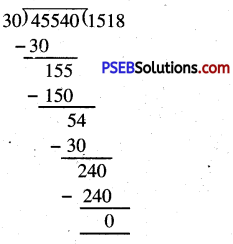# PSEB 5th Class Maths Solutions Chapter 2 Fundamental Operations on Numbers Ex 2.8

Punjab State Board PSEB 5th Class Maths Book Solutions Chapter 2 Fundamental Operations on Numbers Ex 2.8 Textbook Exercise Questions and Answers.

## PSEB Solutions for Class 5 Maths Chapter 2 Fundamental Operations on Numbers Ex 2.8

Question 1.
In a stadium, in the match of cricket there are 84000 people sitting in 24 rows. How many people are sitting in a row?
Solution:
Total number of people = 84000
Number of rows = 24
Number of people sitting in each row
= 84000 ÷ 24
= 3500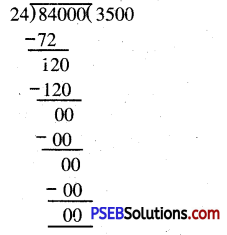Question 2.
You have ₹ 99825 which is to be distributed equally among 33 friends. How much amount will each friend get ?
Solution:
Total amount = ₹ 99825
Number of friends = 33
Each friend will get = ₹ 99825 ÷ 33
= ₹ 3025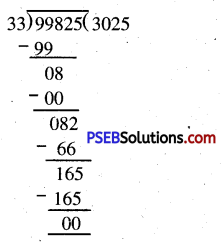Question 3.
My grandfather divided ₹ 72000 equally among four brothers sisters. How much will each get?
Solution:
Total amount = ₹ 72000
Number of brothers and sisters = 4
Each will get
= 72000÷4
= 18000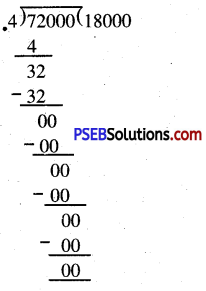Question 4.
What number must be multiplied with 26 to get 14508?
Solution:
Number to be obtained = 14508
The given number = 26
The required number = 14508 ÷ 26
= 558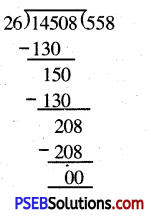Question 5.
The gardener has 23976 flowers to make garlands. One garland has 24 flowers in it. How many garland can be made from 23976 flowers ?
Solution:
Total number of flowers = 23976
Number of flowers in one garland = 24
Total number of garlands = 23976 ÷ 24
= 999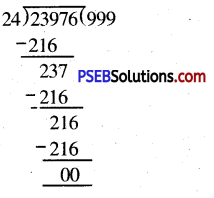Question 6.
How many ₹ 2000 notes are there in forty thousand rupees ?
Solution:
Total amount = ₹ 40,000
Value of one note = ₹ 2000
Number of notes = ₹ 40,000 ÷ ₹ 2000
= 20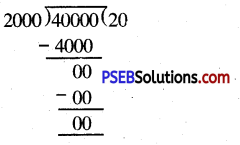Question 7.
I need change of ₹ 25,000. How many following notes shall I get ?
(a) Number of notes of ₹ 1000 = ……….
(b) Number of notes of ₹ 500 = ………..
(c) Number of notes of ₹ 100 = ………..
Solution:
Total amount = ₹ 25,000
(a) Number of ₹ 1000 notes
= ₹ 25000 ÷ ₹ 1000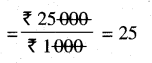(b) Number of ₹ 500 notes
= ₹ 25000 ÷ ₹ 500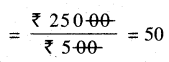(c) Number of ₹ 100 notes
= ₹ 25000 ÷ ₹ 100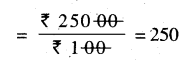Question 8.
A J.C.B. machine picks 900 bricks in a round. How many rounds will it take to pick 99000 bricks ?
Solution:
Total number of bricks = 99,000
Number of bricks picked in one round = 900
Number of rounds
= 99,000 ÷ 900
= $$\frac{99000}{900}$$ = 110

Question 9.
The cost of a railway ticket is ₹ 78. Palak gave ₹ 7722 for buying tickets. How many tickets will she get ?
Solution:
Cost of 1 ticket = ₹ 78
Total amount = ₹ 7722
Number of tickets she will get
= ₹ 7722 ÷ ₹ 78
= 99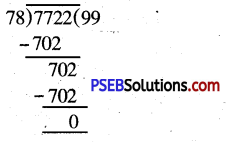Question 10.
A factory manufactures 45540 ice cream cones in the month of June. How many ice cream cones are manufactured in a day ?
Solution:
Total number of ice cream cones manufactured = 45540
Number of the days in the month of June = 30
Number of cones manufactured in one day = 45540 ÷ 30 = 1518